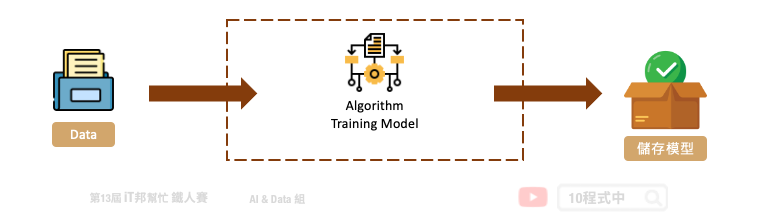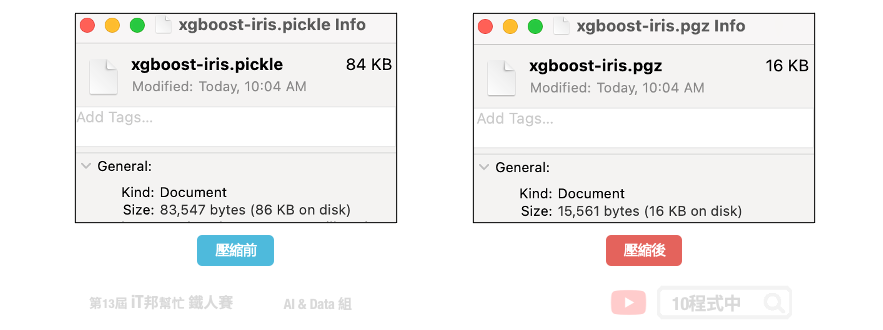DAY 28
0
AI & Data

# 儲存訓練好的模型

## 今日學習目標

• 使用 pickle + gzip 儲存模型
• 將訓練好的模型打包並儲存
• 載入儲存的模型
• 讀取打包好的模型並預測

## 前言## 1) 載入資料集

``````import pandas as pd
import numpy as np
import matplotlib.pyplot as plt
import seaborn as sns

df_data = pd.DataFrame(data= np.c_[iris['data'], iris['target']],
columns= ['SepalLengthCm','SepalWidthCm','PetalLengthCm','PetalWidthCm','Species'])
df_data
``````

## 2) 切割訓練集與測試集

``````from sklearn.model_selection import train_test_split
X = df_data.drop(labels=['Species'],axis=1).values # 移除Species並取得剩下欄位資料
y = df_data['Species'].values
X_train, X_test, y_train, y_test = train_test_split(X, y, test_size=0.3, random_state=42, stratify=y)

print('train shape:', X_train.shape)
print('test shape:', X_test.shape)
``````

## 訓練模型 - XGBoost

XGBoost 模型是目前最熱門的演算法模型之一，詳細的內容可以參考 [Day 15] 機器學習常勝軍 - XGBoost。裡面會有介紹詳細的模型說明與手把手實作。當然大家也可以試著用其他 Sklearn 的模型訓練看看，一樣可以透過 `pickle` 來儲存訓練好的模型。

``````from xgboost import XGBClassifier

# 建立 XGBClassifier 模型
xgboostModel = XGBClassifier(n_estimators=100, learning_rate= 0.3)
# 使用訓練資料訓練模型
xgboostModel.fit(X_train, y_train)
# 使用訓練資料預測分類
predicted = xgboostModel.predict(X_train)
``````

## 儲存 XGboost 模型

### 1. 使用 pickle 儲存模型

``````import pickle
with open('./model/xgboost-iris.pickle', 'wb') as f:
pickle.dump(xgboostModel, f)
``````

### 2. 使用 pickle 儲存模型並利用 gzip 壓縮

``````import pickle
import gzip
with gzip.GzipFile('./model/xgboost-iris.pgz', 'w') as f:
pickle.dump(xgboostModel, f)
``````## 載入 XGboost 模型

### 1. 載入 pickle 格式模型

``````import pickle
import gzip

#讀取Model
with gzip.open('./model/xgboost-iris.pgz', 'r') as f:
pred=xgboostModel.predict(np.array([[5.5, 2.4, 3.7, 1. ]]))
print(pred)
``````

### 2. 載入 gzip 格式模型

``````#讀取Model
with open('./model/xgboost-iris.pickle', 'rb') as f:
pred=xgboostModel.predict(np.array([[5.5, 2.4, 3.7, 1. ]]))
print(pred)
``````